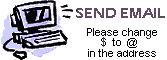Find the next number in each sequence
 W I N I O N O W
Number Series
The Contest Center
Wappingers Falls, NY 12590
 W I N I O N O W

Number Series 1
What are the next two numbers in this series? (Each number determines the next number. You do not need any outside knowledge, like subway stops, the periodic table, sizes of planets, etc.)

1, 4, 8, 13, 21, 30, 36, 45, 54, 63, 73, ?, ?

Number Series 2
What are the next two numbers in this series? (This is a purely arithmetic series, where each number determines the next number.)

30, 33, 42, 50, 55, 80, 88, 152, 162, 174, ?, ?

Number Series 3
What are the 12th, 21st, and 23rd numbers in this series? (Each number is uniquely determined by its position on the list. You do not need any outside knowledge, like the words to a song, the order of states entering the union, etc.)

1, 4, 3, 11, 15, 13, 17, 24, 23, 73, 101, ?, ...

Number Series 4
What are the next two numbers in this series? (Each number is uniquely determined by its position on the list. You will need some knowledge of mathematics for this puzzle.)

3, 5, 11, 17, 29, 41, 59, 71, 101, ?, ?, ...

Number Series 5
What are the next two numbers in this series? (These are successive numbers sharing a common property. No math or outside knowledge is needed.)

3, 39, 41, 43, 45, 49, 51, 53, 55, 64, ?, ?, ...

Number Series 6
What are the next six numbers in this series? (Each number is determined from the number before by a simple mathematical relationship. You will need some math.)

2, 3, 4, 6, 8, 10, 13, 16, 20, 24, ?, ?, ?, ...

Number Series 7
What are the next two numbers in this series? (This series is not mathematical. Some patriotism is required.)

1, 6, 10, 2, 3, 4, 2, 3, 6, 6, 2, 7, ?, ?, ...

Number Series 8
What are the next three numbers in this series? (Each number is determined from the number before by a simple mathematical relationship. You will need some math.)

1, 2, 3, 5, 7, 10, 12, 14, 17, 19, 22, 25, ?, ?, ?, ...Send us an email to submit answers to these puzzles, or to submit new number series puzzles. We welcome new puzzles from our website visitors. Be sure to change the \$ to an @ in our email address.